# Catch up on the latest AI articles# What Are The Desirable Properties For Positional Embedding In BERT?

3main points
✔️ Extensive analysis of the properties and characteristics of positional embedding
✔️
Analyze positional embedding from three metrics: translation invariance, monotonicity, and symmetry
✔️
Experiment and validate the effectiveness of positional embedding in various downstream tasks

On Position Embeddings in BERT
written by Benyou WangLifeng ShangChristina LiomaXin JiangHao YangQun LiuJakob Grue Simonsen
(Submitted on 29 Sept 2020 (modified: 02 Mar 2021))

Subjects: Position Embedding, BERT, pretrained language model.code：## First of all

In the Transformer-based model, Positional Embedding (PE) is used to understand the location information of the input token. There are various settings for this PE, such as absolute/relative position, learnable/fixed.

So what kind of PE should you use?　Or, what properties of PEs will give excellent performance? In the paper presented in this article, three properties, namely translational invariance, monotonicity, and symmetry, are presented as effective indices for the analysis and interpretation of PEs. In addition, comparative experiments of various PEs were conducted to provide answers to the previous questions.

### Properties of Positional Encodings

As for the three properties of positional encoding, the original paper proposes the following three

Characteristics 1. monotonicity

First, let $\phi(⋅,⋅)$ be a function to calculate the proximity between positional embeddings (e.g. inner product). The monotonicity is the property that the farther the two positional embeddings are from each other, the smaller the proximity.

$\forall x,m,n \in N: m＞n \Leftrightarrow \phi(\overset{\rightarrow}{x}, \overset{\longrightarrow}{x+m}) ＜ \phi(\overset{\rightarrow}{x}, \overset{\longrightarrow}{x+n})$

$\underset{x}{\rightarrow}$ represents a positional embedding at position $x$.

Characteristic 2. translation invariance

Translation invariance pertains to the property that the proximity of two positional embeddings whose relative positions are equal is invariant.

$\forall x_1,...,x_n,m \in N: \phi(\overset{\rightarrow}{x_1}, \overset{\longrightarrow}{x_1+m}) = \phi(\overset{\rightarrow}{x_2}, \overset{\longrightarrow}{x_2+m}) = ... = \phi(\overset{\rightarrow}{x_n}, \overset{\longrightarrow}{x_n+m})$

Characteristics 3. symmetry

Symmetry pertains to the fact that the proximity of two positional embeddings has the following properties.

$\forall x,y \in N: phi(\overset{\rightarrow}{x}, \overset{\rightarrow}{y})=phi(\overset{\rightarrow}{y}, \overset{\rightarrow}{x})$

Based on these three properties, we will investigate a variety of positional embeddings.

### Understanding positional embedding based on characteristics

Absolute and relative position embedding (APE/RPE)

There are two types of positional embedding: absolute positional embedding (APE) and relative positional embedding (RPE). In addition to the difference in whether the embedding represents an absolute position ($x \in N$) or a relative position ($x-y, x,y \in N$), there is a difference in the attention calculation mechanism between them, as shown below.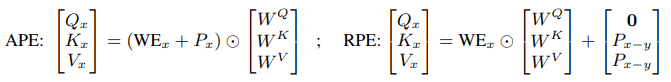$WE_x$ denotes word embedding, $P_x$ denotes absolute positional embedding, and $P_{x-y}$ denotes relative positional embedding. Next, we examine the four existing types of positional embeddings shown below, each based on the aforementioned properties.

In the case of sine wave PE, the dot product between the two position vectors is as follows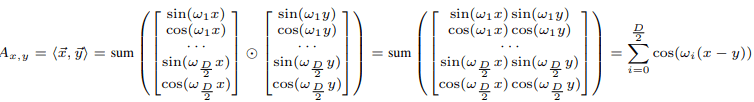In the case of the sine wave PE, the inner product is only related to $x-y$, so both properties 2 (translation invariance) and 3 (symmetry) are satisfied. Property 1 (monotonicity), on the other hand, is equivalent to the monotonicity of the map $\psi(m)=\sum^{D/2}_{i=1}cos(\omega_i m)$. This is monotonic in the interval where the derivative $omega^{\prime}(m)=\sum^{D/2}_{i=1}-\omega_i sin(\omega_i m)$ of $\psi(m)$ does not change sign.

Therefore, the range in which monotonicity is valid depends on the value of $\omega_i$. For example, in the case of $\omega_i=(1/10000)^{2i/D}$, monotonicity is valid in the range of m from 0 to 50, but not in the range beyond that. Therefore, by making this $\omega_i$ learnable, we can adaptively adjust the range of monotonicity.

RPE encodes relative distance instead of absolute position of a word. By its nature, property 2 (translational invariance) holds (if $x_1-y_1=x_2-y_2$, then it is defined to be $P_{x_1-y_1}=P_{x_2-y_2}$).

As for property 3 (symmetry), it is no longer valid when the embedding is different in forward/backward ($P_{i-j} \neq P_{j-i}$). Characteristic 1 (monotonicity) in the case of sinusoidal RPE has local monotonicity depending on the value of $\omega$, as in sinusoidal APE. The difference, however, is that in sinusoidal RPE the dimension is the same as the dimension of each head (64 in BERT), whereas in APE it is 768. Note also that in the case of sinusoidal RPE, if the absolute values of the relative positions are equal, the embedding is the same (regardless of before and after).

## Experiment

To begin, we investigate the properties of the six basic positional embeddings (and their combinations) listed in the following table.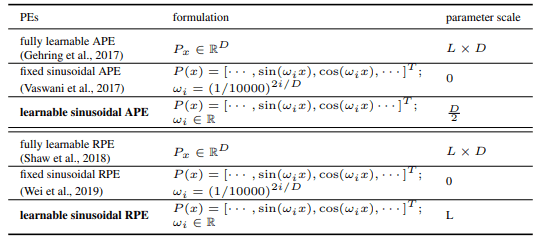A quantitative evaluation of the three aforementioned properties of these positional embeddings is as follows.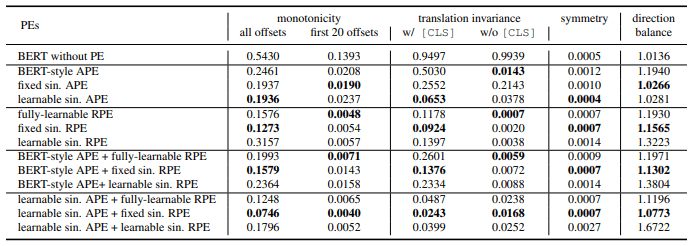### The dot product between position vectors

For APE and RPE, the dot product (which can be regarded as the proximity) between any two position vectors is as follows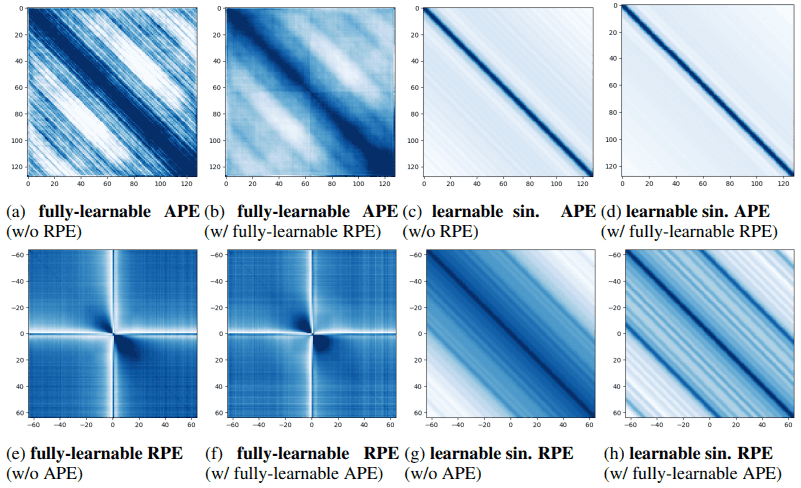In APE, the dot product of adjacent position vectors (e.g., on the diagonal from the top left to the bottom right in (a)) is larger. This tendency is especially pronounced in the case of the learnable sinusoidal APE (c). We can also see that the overall trend does not change much when RPE is added to APE ((b),(d)).

In the fully learnable RPE((e),(f)), there are bright areas in the vertical and horizontal directions. This means that the position embedding when the absolute value of the relative position is small is very different from other relative position vectors. Also, the dark area in the diagonal direction means that the position embedding for the case of the large absolute value of relative position is similar to each other.

In other words, fully learnable RPEs can distinguish between very small absolute values of relative positions, but not very large ones. This may be due to the fact that fully learnable RPEs are particularly concerned with local dependencies.

We compare the performance of PEs on classification and span prediction tasks. Specifically, we perform fine-tuning using GLUE and SQuAD as benchmarks and evaluate the average value of five runs for each dataset.

PE in GLUE

The results in the GLUE benchmark are as follows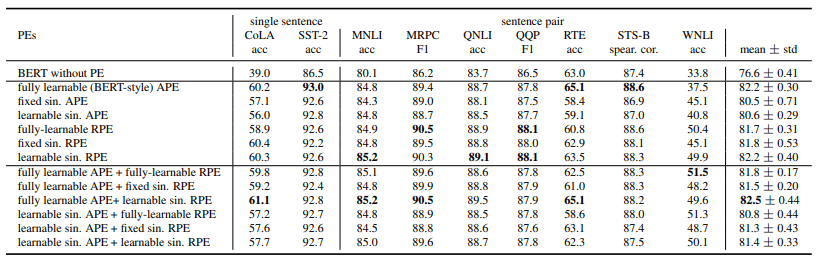On GLUE, the fully learnable APE performs well and shows overall better results than the other cases where only either APE/RPE is used.

Regarding the case of combining APE and RPE, fully learnable APE + learnable sinusoidal RPE is relatively better. We also found that the combination of APE and RPE does not necessarily improve the performance.

The results in the SQuAD benchmark are as follows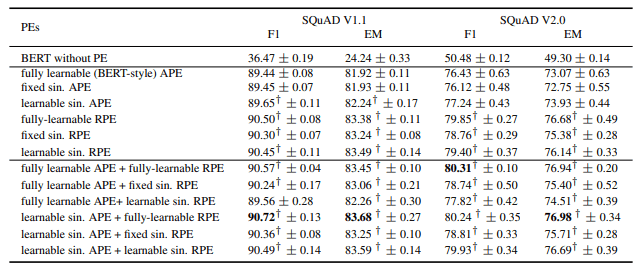On SQuAD, almost all BERT models using RPE significantly outperformed the fully learnable APE. The best performing models in SQuAD V1.1/V2.0 were both those using fully learnable RPE.

These differences in GLUE/SQuAD can be attributed to the relative superiority of absolute position embedding (APE) in handling the [CLS] token, which is important for the classification task (GLUE). In addition, the overall learnable sinusoidal APE/RPE outperforms the fixed sinusoidal APE/RPE, demonstrating the effectiveness of flexibly varying $\omega$. Furthermore, we find that combining APE and RPE can improve performance in some cases.

### The relationship between PE characteristics and tasks

The relevance of the three location embedding properties presented in the introduction to the downstream task is shown in the following table.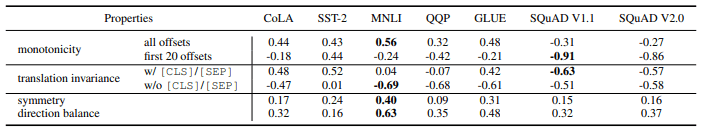A number close to 1 (-1) indicates how positively (negatively) correlated the task performance is with the violation of the characteristic.

Thus, we find that violations of local monotonicity and translational invariance (many negative numbers) are detrimental, while violations of symmetry (many positive numbers) are beneficial. Symmetry leads to difficulty in distinguishing between preceding and following tokens, which appears to be an undesirable property for many tasks.

## Summary

In the paper presented in this article, we defined three metrics for analyzing positional embedding and conducted comparative experiments on various positional embeddings. The results show that breaking translational invariance and monotonicity degrades downstream task performance while breaking contrast improves downstream task performance. We also found that fully learnable absolute position embedding generally improves performance on the classification task, while relative position embedding improves performance on the span prediction task.

Location embedding is an integral part of the highly successful Transformer-based architecture. This work is of great importance as it provides useful insights into positional embedding.

If you have any suggestions for improvement of the content of the article,